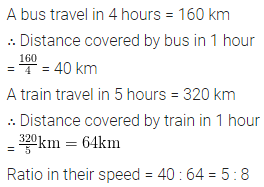# ML Aggarwal Class 6 Solutions for ICSE Maths Chapter 8 Ratio and Proportion Ex 8.3

## ML Aggarwal Class 6 Solutions for ICSE Maths Chapter 8 Ratio and Proportion Ex 8.3

Question 1.
If the cost of 9 m cloth is ₹378, find the cost of 4 m cloth.
Solution: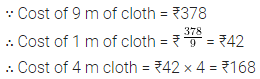Question 2.
The weight of 36 books is 12 kg. What is weight of 75 such books?
Solution:Question 3.
Five pens cost ₹115. How many pens can you buy in ₹207?
Solution: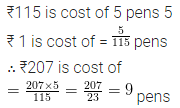Question 4.
A car consumes 8 litres of petrol in covering a distance of 100 km. How many kilometres will it travel in 26 litres of petrol?
Solution:Question 5.
A truck requires 108 litres of diesel for covering a distance of 594 km. How much diesel will be required by the truck to cover a distance of 1650 km?
Solution: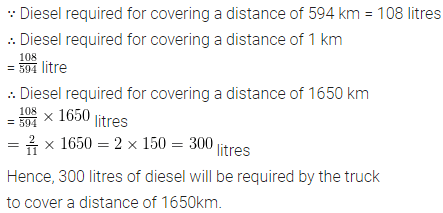Question 6.
A transport company charges ₹5400 to carry 80 quintals of weight. What will it charge to carry 126 quintals of weight (same distance)?
Solution: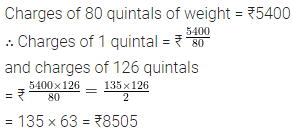Question 7.
42 metres of cloth is required to make 20 shirts of the same size. How much cloth will be required to make 36 shirts of that size?
Solution:Question 8.
Cost of 5 kg of rice is ₹107.50.
(i) What will be the cost of 8 kg of rice?
(ii) What quantity of rice can be purchased in ₹64.5?
Solution:Question 9.
Cost of 4 dozen bananas is ₹ 180. How many bananas can be purchased for ₹37.50?
Solution: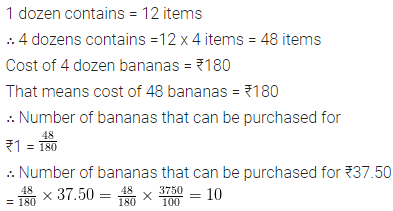Question 10.
Aman purchases 12 pens for ₹156 and Payush buys 9 pens for ₹1108. Can you say who got the pens cheaper?
Solution: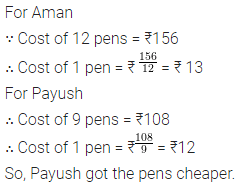Question 11.
Rohit made 42 runs in 6 overs and Virat made 63 runs in 7 overs. Who made more runs per over?
Solution:Question 12.
A bus travels 160 km in 4 hours and a train travels 320 km in 5 hours at uniform speeds, then find the ratio of the distance travelled by them in one hour.
Solution: